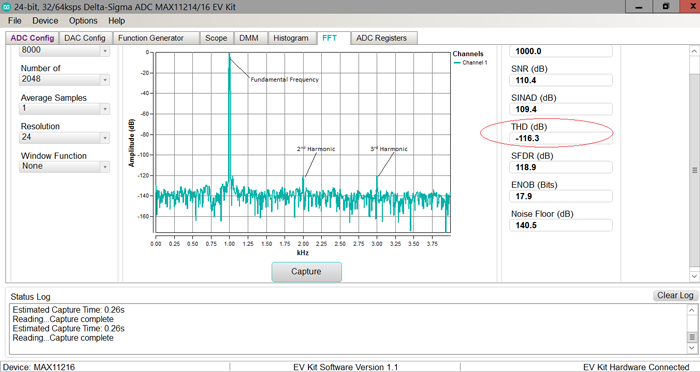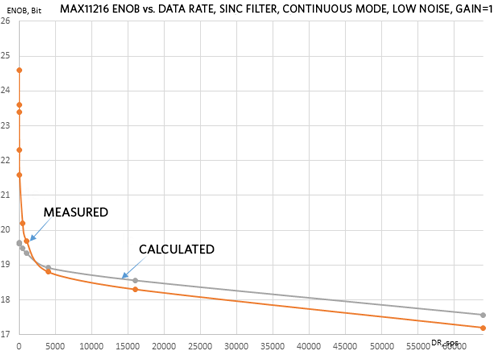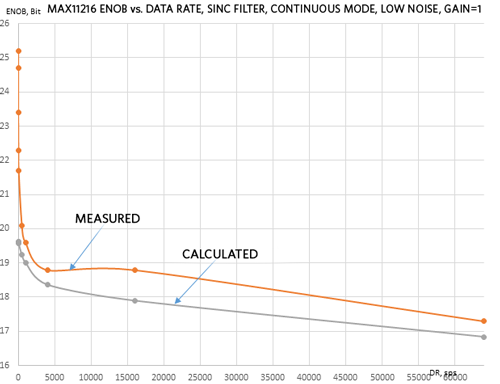# How to Calculate ENOB for ADC Dynamic Performance Measurement

### Abstract

SNR, SINAD, THD, and ENOB values are common measures of the dynamic performance of ADCs, and ENOB can be calculated directly from the known values of SNR and THD.

### Introduction

The dynamic performance of an analog-to-digital converter (ADC) is determined by the effective number of bits (ENOB). In this application note, we examine the relationship of ENOB with other dynamic characteristics of ADCs such as signal-to-noise ratio (SNR), signal-to-noise and distortion ratio (SINAD), and total harmonic distortion (THD). We also compare the theoretically calculated ENOB of the MAX11216 24-bit high performance delta-sigma ADC to the measured value obtained in the lab.

### How Are SNR, SINAD, THD, and ENOB Related?

#### Signal-to-Noise Ratio

Signal-to-noise ratio (SNR) is the fundamental frequency signal power level (PS) to the noise power level (PN) ratio and is mathematically expressed in Equation 1.

SNR = 10log(PS/PN) = 10logPS – 10logPN.

The ideal theoretical SNR is calculated directly from the resolution (N bit) as SNR = (6.02 × N + 1.76)dB. However, for delta-sigma ADCs like the MAX11216, which features internal programmable low-pass digital filters, the SNR can be increased by adjusting the amount of filtering. Filter more by reducing the filter bandwidth for a higher SNR, and filter less by increasing the filter bandwidth for a higher data rate.

Figure 1 shows a fast Fourier transform (FFT) of the MAX11216 with continuous mode, sinc filter, and buffer features. PS is the fundamental frequency signal power level and PN is the noise power level, resulting in an SNR of 110.4dB at a data rate of 8Ksps. FFT is an analog spectrum analyzer that measures the amplitude of the fundamental frequency and its various harmonics as well as the non-harmonic spurious and noise components of a digitized signal.#### Signal-to-Noise and Distortion Ratio

Signal-to-noise and distortion ratio (SINAD) is the fundamental frequency signal power level (PS) to the noise plus distortion power level (PN+D) ratio. SINAD is mathematically expressed as in equation 2.

SINAD = 10log[PS/(PN+D] = 10logPS – 10logPN+D.

Distortion includes the harmonics and spurious as shown in Figure 2 of a MAX11216 FFT with continuous mode, sinc filter, and buffer features and SINAD = 109.4dB at a data rate of 8Ksps.#### Total Harmonic Distortion

Total harmonic distortion (THD) is the ratio of the fundamental signal power level to the power of the sum of its harmonics excluding noise. Generally, the first five harmonics contribute to the most distortion. Hence, in calculating THD, only the first five harmonics are used as shown in Equation 3.

THD (dB) = 10log(PS) – 10log(P2 + P3 + P4 + P5 + P6)

where PS and P2 to P6 are in mW.

For example, if PS = P1 = 1mW, P2 = 0.1nW, P3 = 0.01nW, P4 = 0.001nW, P5 = 0.0001nW, and P6 = 0.00001nW, then the THD is calculated as follows:

THD(dB) = 10log(1) – 10log[(0.1 + 0.01 + 0.001 + 0.0001 + 0.00001) × 10−6]
THD(dB) = −69.5074dB

If the sixth harmonic of 0.00002nW was counted, the THD would increase to −69.5070dB, which is very insignificant.

Figure 3 shows the MAX11216 with a THD of -116.3dB, an input frequency of 1KHz, a sample rate of 8Ksps, and continuous mode, sinc filter, and buffer features.### Effective Number of Bits

The effective number of bits (ENOB) is the number of bits when both noise and distortion are considered and is mathematically expressed in Equation 4.

To express ENOB in terms of SNR and THD, see the following calculations:

1. Use Equation 2 and Equation 1 as follows to determine Equation 5:
SINAD = 10log[PS/(PN+D] = 10logPS – 10logPN+D.
SNR = 10log (PS/PN)
log (PS/PN) = SNR/10
PS/PN = 10SNR/10
PN/PS = 10−SNR/10
2. Equation 5 can be also expressed as follows:
PD/PS = 10−THD/10
3. Add Equation 5 and Equation 6 to determine Equation 7.
(PN+PD)/PS = 10−SNR/10 + 10−THD/10
PS/(PN+D) = 1/(10−SNR/10 + 10−THD/10) = (10−SNR/10 + 10-THD/10)−1
4. Substitute Equation 7 into Equation 2.
= −10log(10−SNR/10 + 10-THD/10)
5. Finally, substitute Equation 8 into Equation 4 to obtain the ENOB equation in terms of SNR and THD as follows:
ENOB = {[−10log(10−SNR/10 + 10−THD/10)] – 1.76}/6.02

### MAX11216 ENOB

Table 1 lists the simulated MAX11216 SNR values for different data rates with a sinc digital filter where VIN = 0V, VAVDD = 3.6V, VAVSS = 0V, VREF = 3.6V, and TA = +25°C.

Table 1. Simulated Continuous Mode SNR (dB) Versus Data Rate and PGA Gain with Sinc Filter
Data Rate (sps) Buffer Gain = 1 Gain = 8 Gain = 128
LN LN LN
1.9 139.8 136.5 140.5 128
3.9 139.4 135.3 139.2 125.4
7.8 139.2 134.7 137.9 122.5
31.2 135.8 132.1 134.3 116.5
62.5 133.8 129.4 131.5 114
500 125.8 121.2 123.3 104.8
1000 123.1 118.5 120.4 101.8
4000 117.7 113.1 115.1 96.5
16000 114.6 109.9 112 93.4
64000 107.8 103.2 105.8 88.2

Table 2 lists the calculated ENOB values using Equation 9 based on the SNR values where THD = 120dB (typical specification in the MAX11216 data sheet), VIN = 0V, VAVDD = 3.6V, VAVSS = 0V, VREF = 3.6V, and TA = +25°C.

Table 2. Calculated Continuous Mode ENOB (Bits) Versus Data Rate and PGA Gain with Sinc Filter
Data Rate (sps) ENOB
Buffer Gain = 1, Low Noise Gain = 8, Low Noise Gain = 128, Low Noise
1.9 19.6336811 19.62522357 19.63479484 19.53506307
3.9 19.6329602 19.62021353 19.63257437 19.45838575
7.8 19.63257437 19.61715616 19.62958982 19.3193091
31.2 19.62246601 19.59803115 19.61487866 18.79339941
62.5 19.6117322 19.56278581 19.59185023 18.4828525
500 19.4727366 19.23395095 19.3643726 17.09481505
1000 19.35361828 19.00585701 19.17360407 16.60710288
4000 18.92511435 18.36098144 18.62499223 15.73432624
16000 18.56137578 17.89619095 18.20615941 15.22101479
64000 17.57240744 16.83558105 17.25547296 14.35832751

Table 3 lists the measured ENOB values.

Table 3. Measured Continuous Mode ENOB (Bits) Versus Data Rate and PGA Gain with Sinc Filter
Data Rate (sps) Buffer Gain = 1 Gain = 8 Gain = 128
LN LN LN
1.9 24.6 25.2 24.8 24.5
3.9 23.4 24.7 23.9 24.4
7.8 23.6 23.4 23.3 23.1
31.2 22.3 22.3 22.1 22
62.5 21.6 21.7 21.5 21.4
500 20.2 20.1 20.2 20
1000 19.7 19.6 19.5 19.3
4000 18.8 18.8 18.7 18.5
16000 18.3 18.8 18.5 18.6
64000 17.2 17.3 17.3 17.3

Figure 4, Figure 5, Figure 6, and Figure 7 compare the measured and calculated ENOB values for the buffer, gain = 1, gain = 8, and gain = 128.Figure 8 shows the measured ENOB values versus the data rates for the buffer and various PGA gains.### Conclusion

The SNR, SINAD, THD, and ENOB values are common measures of the dynamic performance of ADCs. The ENOB can be easily and accurately calculated based on the signal-to-noise ratio (SNR) and the total harmonic distortion (THD). The MAX11216 ENOB values obtained in the lab confirm that the measured data matches closely with the values calculated based on the ENOB equation. For delta-sigma ADCs with internal programmable digital filters, the measured data also confirms that increasing the amount of filtering increases the SNR and ENOB.